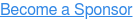# Chief Data & Analytics Officers, Insurance

September 15-16, 2020 | DoubleTree by Hilton Hotel, Tarrytown, New Yorktrue true true true true true true true true true true true true true true true true true true true true true true true true true true true true true true true true true true true true true true true true true true true true true true true true true true true true true true true true true true true true true true true true true true true true true true true true true true true true true true true